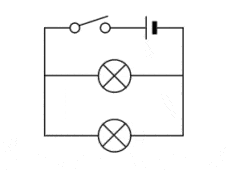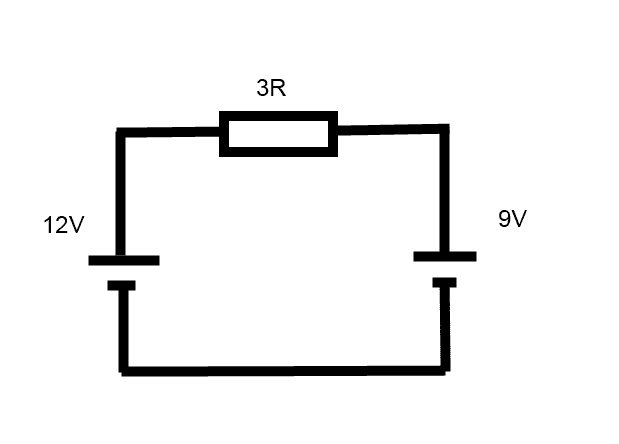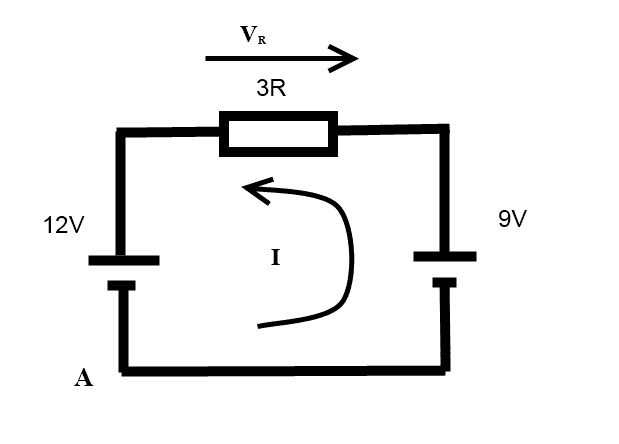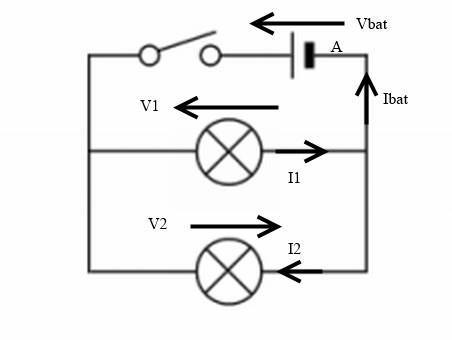# Kirchoff's Voltage Law

• TiernanW
The left hand battery is 12V and the right hand 9V so it would be...The left hand battery is 12 - 9 = 3V and the right hand 9 - 12 = 4V so the total voltage drop across the resistor is 9 - 4 = 5V.In summary, the current through the bulbs would be going in the same direction given the topology you are presenting however in one video I seen for Kirchoff's Laws (Sorry can't link to it forgot what its called), the guy took the bottom 4 sided network (in this case with the 2 bulbs) and the current went round in a square all in the same direction, so that would mean that it flows to the right through the

## Homework StatementWhat way does the current flow through the first light-bulb?

## The Attempt at a Solution

This isn't really a homework question but related ot my school work. I'm doing Kirchoff's Laws and normally I would have just thought that the current (if we use NEGATIVE to POSITIVE) down the right-hand side and then goes left through each bulb flowing back towards the switch. However in one video I seen for Kirchoff's Laws (Sorry can't link to it forgot what its called), the guy took the bottom 4 sided network (in this case with the 2 bulbs) and the current went round in a square all in the same direction, so that would mean that it flows to the right through the first bulb. That confused me a little bit. What is the correct way?

Conventional Flow assumes that current flows out of the positive terminal, through the circuit and into the negative terminal of the source.
Electron Flow is the actual flow of current, where electrons flow out of the negative terminal, through the circuit and into the positive terminal of the source.

Conventional flow of current is what was chosen back when electricity was dscovered. They are both used in literature.

QuantumQuest said:
Conventional Flow assumes that current flows out of the positive terminal, through the circuit and into the negative terminal of the source.
Electron Flow is the actual flow of current, where electrons flow out of the negative terminal, through the circuit and into the positive terminal of the source.

Conventional flow of current is what was chosen back when electricity was dscovered. They are both used in literature.
Sorry, yes I know. I just meant the guy took that little network out and hand everything flowing clockwise through it, but if I followed that circuit, I would have though the current would have went through the top bulb going to the left and the same with the other bulb. But when he took it out he had the current flowing through the top bulb going to the right and the bottom bulb had it going through to the left so it was all clockwise, but I didn't think it would be clock wise because the current going through top bulb would be going to the left. Hope that made sense. xD

I just meant the guy took that little network out and hand everything flowing clockwise through it...
What do you mean "took out" the bottom network with the two bulbs? If you do this you have no source and thus no current.

TiernanW said:
Sorry, yes I know. I just meant the guy took that little network out and hand everything flowing clockwise through it, but if I followed that circuit, I would have though the current would have went through the top bulb going to the left and the same with the other bulb. But when he took it out he had the current flowing through the top bulb going to the right and the bottom bulb had it going through to the left so it was all clockwise, but I didn't think it would be clock wise because the current going through top bulb would be going to the left. Hope that made sense. xD
the current through the bulbs would be going in the same direction given the topology you are presenting

TiernanW said:
However in one video I seen for Kirchoff's Laws (Sorry can't link to it forgot what its called), the guy took the bottom 4 sided network (in this case with the 2 bulbs) and the current went round in a square all in the same direction, so that would mean that it flows to the right through the first bulb. That confused me a little bit. What is the correct way?

It's impossible to speculate what the man in that unknown video was doing but perhaps this will help...

In general when you apply Kirchoff's Laws to solve a problem the first thing you do is mark up the drawing with arrows showing the direction of +ve current. It's not always obvious which direction current is flowing but that doesn't matter. You can mark up the circuit with current arrows in an arbitrary way just as long as long as you are consistent when you come to mark up the voltage drops. The next step is usually to write and solve a bunch of simultaneous equations to get values for all the voltage drops and currents. If one of the currents turns out to be -ve that means your earlier assumption about the direction of the current was wrong (wrong isn't quite the right word but it will do).

For example consider this circuit with two batteries and a resistor.The left hand battery is 12V and the right hand 9V so it would be reasonable to assume that current would flow from left to right through the resistor (eg clockwise).

However for the moment I will pretended that it's not obvious which direction current flows and will define +ve current as anti-clockwise as shown below. To be consistent with my assumption that current flows anti clockwise I also have to define the direction of the voltage drop across the resistor VR as shown on the drawing. That also "looks wrong" but it must be consistent with the direction I assumed the current was flowing.Now we apply KVL...

I'll start at point A and go around clockwise (again it's an arbitrary choice).

+12V +VR + (-9V) = 0

Solve to give
VR = -3V

Oh look, the voltage is negative which means the voltage across the resistor is actually the other way to my original assumption.

Then applying Ohms law
I = VR/R = -3/3 = -1A

Oh look the current is negative which means the current is actually flowing in the opposite direction to my original assumption.

The point is that it doesn't matter which way you assume current is flowing as long as you are consistent. In the end it all falls out in the wash.

•TiernanW
Based on your OP I have marked up your circuit in the way it appears the man in the unknown video might have done. Note that I have defined conventional +ve current as flowing from left to right in the top bulb and right to left in the lower bulb.Applying KVL around top loop starting at A and going anticlockwise. ..

+Vbat + (-V1) = 0
so
V1 = Vbat

KVL around outer loop...

+Vbat + (V2) = 0
so
V2 = -Vbat

So actually the left hand side of both bulbs is +ve.

Using Ohms law where R is the bulb resistance...

I1 = V1/R = Vbat/R
This is +ve so the current through the top bulb does flow in the direction shown.

I2 = V2/R = -Vbat/R
This is -ve so the current through the lower bulb flow in the opposite direction to that shown.

So conventional +ve current flows through both bulbs left to right.

•TiernanW